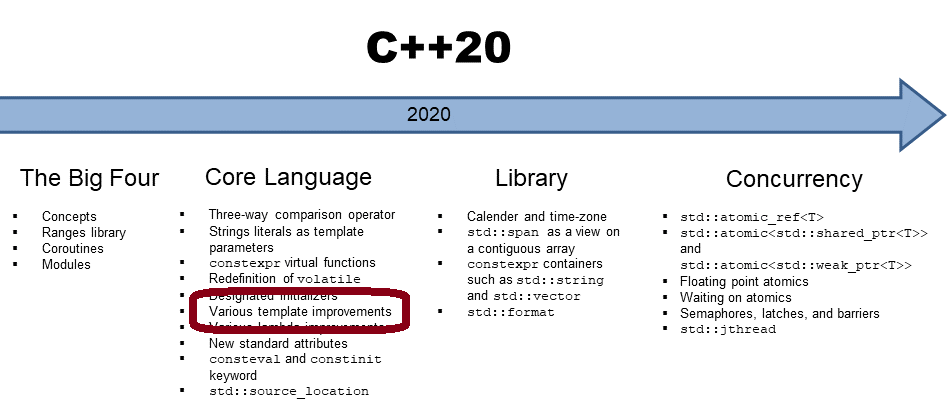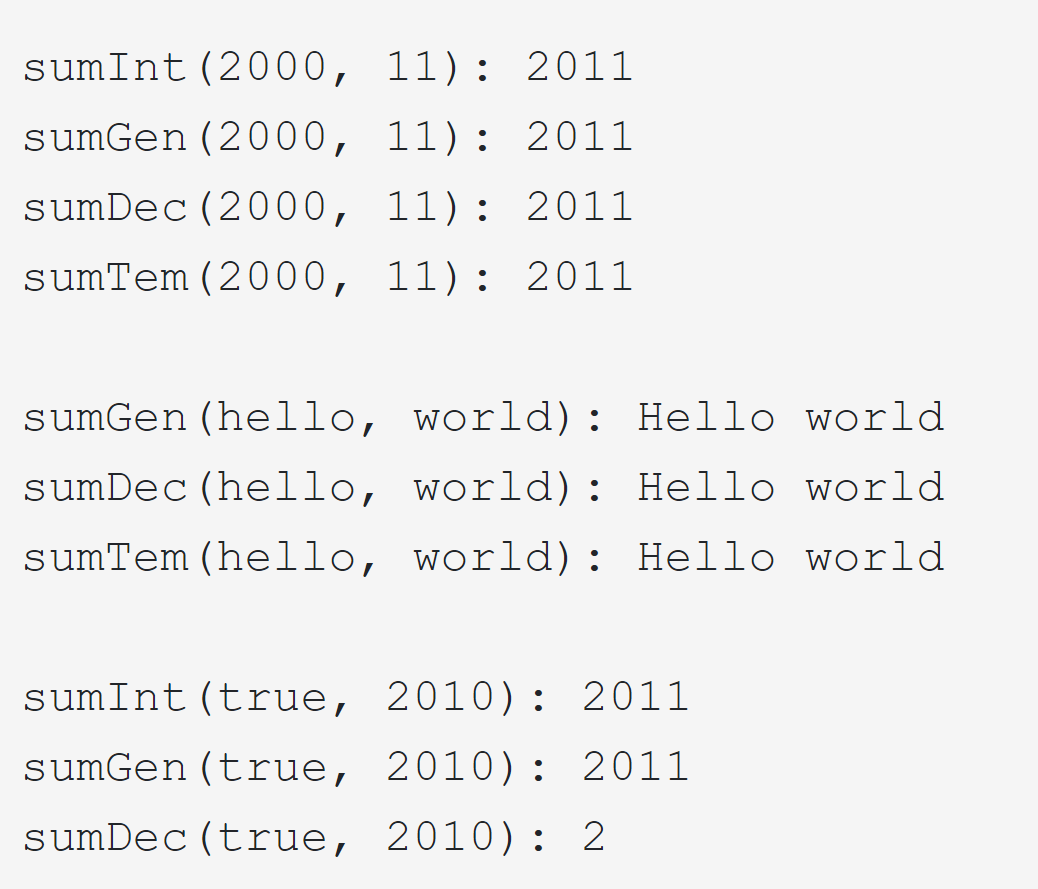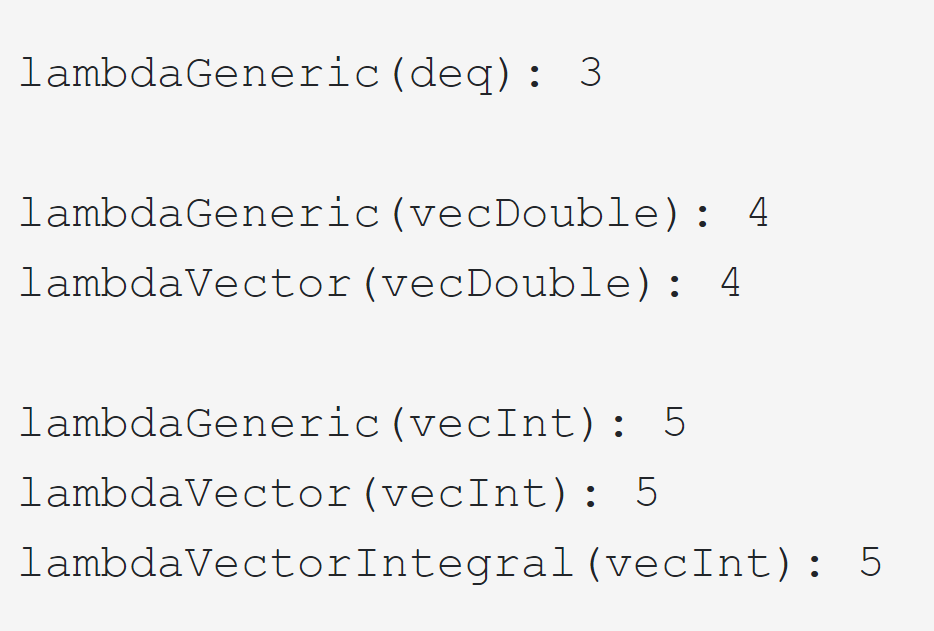# More Powerful Lambdas with C++20

Thanks to C++20, lambdas have become more powerful. From the various lambda improvements, template parameters for lambdas are my favorite ones.Lambdas support with C++20 template parameters can be default-constructed and support copy-assignment when they have no state and can be used in unevaluated contexts. Additionally, they detect when you implicitly copy the this pointer. This means a significant cause of undefined behavior with lambdas is gone.

## Template Parameter for Lambdas

Admittedly, the differences between typed lambdas, generic lambdas, and template lambdas (template parameters for lambdas) are subtle.

### Four lambda variations

The following program presents four variations of the add function using lambdas for their implementation.

##Modernes C++ Mentoring

Be part of my mentoring programs:

• "Fundamentals for C++ Professionals" (open)
• "Design Patterns and Architectural Patterns with C++" (open)
• "C++20: Get the Details" (reopens December 2023)
• Do you want to stay informed about my mentoring programs: Subscribe via E-Mail.

```// templateLambda.cpp

#include <iostream>
#include <string>
#include <vector>

auto sumInt = [](int fir, int sec) { return fir + sec; };            // only to int convertible types    (C++11)
auto sumGen = [](auto fir, auto sec) { return fir + sec; };          // arbitrary types                  (C++14)
auto sumDec = [](auto fir, decltype(fir) sec) { return fir + sec; }; // arbitrary, but convertible types (C++14)
auto sumTem = []<typename T>(T fir, T sec) { return fir + sec; };    // arbitrary, but identical types   (C++20)

int main() {

std::cout << std::endl;
// (1)
std::cout << "sumInt(2000, 11): " << sumInt(2000, 11) << std::endl;
std::cout << "sumGen(2000, 11): " << sumGen(2000, 11) << std::endl;
std::cout << "sumDec(2000, 11): " << sumDec(2000, 11) << std::endl;
std::cout << "sumTem(2000, 11): " << sumTem(2000, 11) << std::endl;

std::cout << std::endl;
// (2)
std::string hello = "Hello ";
std::string world = "world";
// std::cout << "sumInt(hello, world): " << sumInt(hello, world) << std::endl; ERROR
std::cout << "sumGen(hello, world): " << sumGen(hello, world) << std::endl;
std::cout << "sumDec(hello, world): " << sumDec(hello, world) << std::endl;
std::cout << "sumTem(hello, world): " << sumTem(hello, world) << std::endl;

std::cout << std::endl;
// (3)
std::cout << "sumInt(true, 2010): " << sumInt(true, 2010) << std::endl;
std::cout << "sumGen(true, 2010): " << sumGen(true, 2010) << std::endl;
std::cout << "sumDec(true, 2010): " << sumDec(true, 2010) << std::endl;
// std::cout << "sumTem(true, 2010): " << sumTem(true, 2010) << std::endl; ERROR

std::cout << std::endl;

}
```

Before I show the presumably astonishing output of the program, I want to compare the four lambdas.

• sumInt
• C++11
• typed lambda
• accepts only to int convertible type
• sumGen
• C++14
• generic lambda
• accepts all types
• sumDec
• C++14
• generic lambda
• the second type must be convertible to the first type
• sumTem
• C++20
• template lambda
• the first type and the second type must be the same

What does this mean for template arguments with different types? Of course, each lambda accepts int‘s (1), and the typed lambda sumInt does not accept strings (2).

Invoking the lambdas with the bool true and the int 2010 may be surprising (3).

• sumInt returns 2011 because true is integral promoted to int.
• sumGen returns 2011 because true is integral promoted to int. There is a subtle difference between sumInt and sumGen, which I present in a few lines.
• sumDec returns 2. Why? The type of the second parameter sec becomes the type of the first parameter fir: thanks to (decltype(fir) sec), the compiler deduces the type of fir and makes it to the type of sec. Consequently, 2010 is converted to true. In the expression fir + sec, fir is integral promoted to 1. Finally, the result is 2.
• sumTem is not valid.

Thanks to the Compiler Explorer and GCC, here is the program’s output.There is an exciting difference between sumInt and sumGen. The integral promotion of the true value happens in the case of sumInt on the caller side. Still, the integral promotion of the true value happens in the case of sumGen in the arithmetic expression fir + sec. Here is the essential part of the program once more

```auto sumInt = [](int fir, int sec) { return fir + sec; };
auto sumGen = [](auto fir, auto sec) { return fir + sec; };

int main() {

sumInt(true, 2010);
sumGen(true, 2010);

}
```

When I use the code snippet in C++ Insights, it shows the difference. I only show the crucial part of the compiler-generated code.

```class __lambda_1_15
{
public:
inline /*constexpr */ int operator()(int fir, int sec) const
{
return fir + sec;
}

};

__lambda_1_15 sumInt = __lambda_1_15{};

class __lambda_2_15
{
public:
template<class type_parameter_0_0, class type_parameter_0_1>
inline /*constexpr */ auto operator()(type_parameter_0_0 fir, type_parameter_0_1 sec) const
{
return fir + sec;
}

#ifdef INSIGHTS_USE_TEMPLATE
template<>
inline /*constexpr */ int operator()(bool fir, int sec) const
{
return static_cast<int>(fir) + sec;                  // (2)
}
#endif

};

__lambda_2_15 sumGen = __lambda_2_15{};

int main()
{
sumInt.operator()(static_cast<int>(true), 2010);       // (1)
sumGen.operator()(true, 2010);
}
```

I assume you know that the compiler generates a function object from a lambda. If you don’t know, Andreas Fertig wrote a few posts on my blog about his tool C++ Insights. One post is about lambdas: C++ Insights posts.

When you carefully study the code snippet, you see the difference. sumInt performs the integral promotion on the call side (1), but sumGen does it in the arithmetic expressions (2).

Honestly, this example was enlightening for me and, hopefully, for you. A more typical use case for template lambdas is the usage of containers in lambdas.

### Template Parameters for Containers

The following program presents lambdas accepting a container. Each lambda returns the size of the container.

```// templateLambdaVector.cpp

#include <concepts>
#include <deque>
#include <iostream>
#include <string>
#include <vector>

auto lambdaGeneric = [](const auto& container) { return container.size(); };
auto lambdaVector = []<typename T>(const std::vector<T>& vec) { return vec.size(); };
auto lambdaVectorIntegral = []<std::integral T>(const std::vector<T>& vec) { return vec.size(); };

int main() {

std::cout << std::endl;

std::deque deq{1, 2, 3};                     // (1)
std::vector vecDouble{1.1, 2.2, 3.3, 4.4};   // (1)
std::vector vecInt{1, 2, 3, 4, 5};           // (1)

std::cout << "lambdaGeneric(deq): " << lambdaGeneric(deq) << std::endl;
// std::cout << "lambdaVector(deq): " << lambdaVector(deq) << std::endl;                  ERROR
// std::cout << "lambdaVectorIntegral(deq): " << lambdaVectorIntegral(deq) << std::endl;  ERROR

std::cout << std::endl;

std::cout << "lambdaGeneric(vecDouble): " << lambdaGeneric(vecDouble) << std::endl;
std::cout << "lambdaVector(vecDouble): " << lambdaVector(vecDouble) << std::endl;
// std::cout << "lambdaVectorIntegral(vecDouble): " << lambdaVectorIntegral(vecDouble) << std::endl;

std::cout << std::endl;

std::cout << "lambdaGeneric(vecInt): " << lambdaGeneric(vecInt) << std::endl;
std::cout << "lambdaVector(vecInt): " << lambdaVector(vecInt) << std::endl;
std::cout << "lambdaVectorIntegral(vecInt): " << lambdaVectorIntegral(vecInt) << std::endl;

std::cout << std::endl;

}
```

lambdaGeneric can be invoked with any data type with a member function size(). lambdaVector is more specific: it only accepts a std::vector. lambdaVectorIntegral uses C++20 concept std::integral. Consequently, it accepts only a std::vector using integral types such as int. To use it, I have to include the header <concepts>. I assume the small program is self-explanatory.There is one feature in the program templateLambdaVector.cpp, that you have probably missed. Since C++17, the compiler can deduce the class template type from its arguments (1). Consequently, instead of the verbose std::vector<int> myVec{1, 2, 3}, you can write std::vector myVec{1, 2, 3}.

## What’s next?

My next post will be about the remaining lambda improvements in C++20.

Thanks a lot to my Patreon Supporters: Matt Braun, Roman Postanciuc, Tobias Zindl, G Prvulovic, Reinhold Dröge, Abernitzke, Frank Grimm, Sakib, Broeserl, António Pina, Sergey Agafyin, Андрей Бурмистров, Jake, GS, Lawton Shoemake, Jozo Leko, John Breland, Venkat Nandam, Jose Francisco, Douglas Tinkham, Kuchlong Kuchlong, Robert Blanch, Truels Wissneth, Kris Kafka, Mario Luoni, Friedrich Huber, lennonli, Pramod Tikare Muralidhara, Peter Ware, Daniel Hufschläger, Alessandro Pezzato, Bob Perry, Satish Vangipuram, Andi Ireland, Richard Ohnemus, Michael Dunsky, Leo Goodstadt, John Wiederhirn, Yacob Cohen-Arazi, Florian Tischler, Robin Furness, Michael Young, Holger Detering, Bernd Mühlhaus, Matthieu Bolt, Stephen Kelley, Kyle Dean, Tusar Palauri, Dmitry Farberov, Juan Dent, George Liao, Daniel Ceperley, Jon T Hess, Stephen Totten, Wolfgang Fütterer, Matthias Grün, Phillip Diekmann, Ben Atakora, Ann Shatoff, Rob North, Bhavith C Achar, and Marco Parri Empoli.

Thanks, in particular, to Jon Hess, Lakshman, Christian Wittenhorst, Sherhy Pyton, Dendi Suhubdy, Sudhakar Belagurusamy, Richard Sargeant, Rusty Fleming, John Nebel, Mipko, Alicja Kaminska, Slavko Radman, and David Poole.

 My special thanks to EmbarcaderoMy special thanks to PVS-StudioMy special thanks to Tipi.buildMy special thanks to Take Up Code## Seminars

I’m happy to give online seminars or face-to-face seminars worldwide. Please call me if you have any questions.

### Standard Seminars (English/German)

Here is a compilation of my standard seminars. These seminars are only meant to give you a first orientation.

• C++ – The Core Language
• C++ – The Standard Library
• C++ – Compact
• C++11 and C++14
• Concurrency with Modern C++
• Design Pattern and Architectural Pattern with C++
• Embedded Programming with Modern C++
• Generic Programming (Templates) with C++

#### New

• Clean Code with Modern C++
• C++20

### Contact Me

Modernes C++ Mentoring,Tags:
0 replies